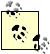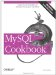# Performing Leap Year Calculations

5.28.1 Problem

You need to perform a date calculation that must account for leap years. For example, the length of a month or a year depends on knowing whether or not the date falls in a leap year.

5.28.2 Solution

Know how to test whether or not a year is a leap year and factor the result into your calculation.

5.28.3 Discussion

Date calculations are complicated by the fact that months don't all have the same number of days, and an additional headache is that February has an extra day during leap years. This section shows how to determine whether or not any given date falls within a leap year, and how to take leap years into account when determining the length of a year or month.

5.28.4 Determining Whether a Date Occurs in a Leap Year

To determine whether or not a date d falls within a leap year, obtain the year component using YEAR( ) and test the result. The common rule-of-thumb test for leap years is "divisible by four," which you can test using the % modulo operator like this:

`YEAR(d) % 4 = 0`

However, that test is not technically correct. (For example, the year 1900 is divisible by four, but is not a leap year.) For a year to qualify as a leap year, it must satisfy both of the following constraints:

• The year must be divisible by four.
• The year cannot be divisible by 100, unless it is also divisible by 400.

The meaning of the second constraint is that turn-of-century years are not leap years, except every fourth century. In SQL, you can express these conditions as follows:

`(YEAR(d) % 4 = 0) AND ((YEAR(d) % 100 != 0) OR (YEAR(d) % 400 = 0))`

Running our date_val table through both the rule-of-thumb leap-year test and the complete test produces the following results:

```mysql> SELECT
-> d,
-> YEAR(d) % 4 = 0
-> AS "rule-of-thumb test",
-> (YEAR(d) % 4 = 0) AND ((YEAR(d) % 100 != 0) OR (YEAR(d) % 400 = 0))
-> AS "complete test"
-> FROM date_val;
+------------+--------------------+---------------+
| d | rule-of-thumb test | complete test |
+------------+--------------------+---------------+
| 1864-02-28 | 1 | 1 |
| 1900-01-15 | 1 | 0 |
| 1987-03-05 | 0 | 0 |
| 1999-12-31 | 0 | 0 |
| 2000-06-04 | 1 | 1 |
+------------+--------------------+---------------+```

As you can see, the two tests don't always produce the same result. In particular, the rule-of-thumb test fails for the year 1900; the complete test result is correct because it accounts for the turn-of-century constraint.Because the complete leap-year test needs to check the century, it requires four-digit year values. Two-digit years are ambiguous with respect to the century, making it impossible to assess the turn-of-century constraint.

If you're working with date values within a program, you can perform leap-year tests with your API language rather than at the SQL level. Pull off the first four digits of the date string to get the year, then test it. If the language performs automatic string-to-number conversion of the year value, this is easy. Otherwise, you must convert the year value to numeric form before testing it.

In Perl and PHP, the leap-year test syntax is as follows:

```\$year = substr (\$date, 0, 4);
\$is_leap = (\$year % 4 == 0) && (\$year % 100 != 0 || \$year % 400 == 0);```

The syntax for Python is similar, although a type conversion operation is necessary:

```year = int (date[0:4])
is_leap = (year % 4 == 0) and (year % 100 != 0 or year % 400 == 0)```

Type conversion is necessary for Java as well:

```int year = Integer.valueOf (date.substring (0, 4)).intValue ( );
boolean is_leap = (year % 4 == 0) && (year % 100 != 0 || year % 400 == 0);```

5.28.5 Using Leap Year Tests for Year-Length Calculations

Years are usually 365 days long, but leap years have an extra day. To determine the length of a year in which a date falls, you can use one of the leap year tests just shown to figure out whether to add a day:

```\$year = substr (\$date, 0, 4);
\$is_leap = (\$year % 4 == 0) && (\$year % 100 != 0 || \$year % 400 == 0);
\$days_in_year = (\$is_leap ? 366 : 365);```

Another way to compute a year's length is to compute the date of the last day of the year and pass it to DAYOFYEAR( ):

```mysql> SET @d = '2003-04-13';
mysql> SELECT DAYOFYEAR(DATE_FORMAT(@d,'%Y-12-31'));
+---------------------------------------+
| DAYOFYEAR(DATE_FORMAT(@d,'%Y-12-31')) |
+---------------------------------------+
| 365 |
+---------------------------------------+
mysql> SET @d = '2004-04-13';
mysql> SELECT DAYOFYEAR(DATE_FORMAT(@d,'%Y-12-31'));
+---------------------------------------+
| DAYOFYEAR(DATE_FORMAT(@d,'%Y-12-31')) |
+---------------------------------------+
| 366 |
+---------------------------------------+```

5.28.6 Using Leap Year Tests for Month-Length Calculations

Earlier in Recipe 5.23, we discussed how to determine the number of days in a month using date shifting to find the last day of the month. Leap-year testing provides an alternate way to accomplish the same objective. All months except February have a fixed length, so by examining the month part of a date, you can tell how long it is. You can also tell how long a given February is if you know whether or not it occurs within a leap year.

A days-in-month expression can be written in SQL like this:

```mysql> SELECT d,
-> ELT(MONTH(d),
-> 31,
-> IF((YEAR(d)%4 = 0) AND ((YEAR(d)%100 != 0) OR (YEAR(d)%400 = 0)),29,28),
-> 31,30,31,30,31,31,30,31,30,31)
-> AS 'days in month'
-> FROM date_val;
+------------+---------------+
| d | days in month |
+------------+---------------+
| 1864-02-28 | 29 |
| 1900-01-15 | 31 |
| 1987-03-05 | 31 |
| 1999-12-31 | 31 |
| 2000-06-04 | 30 |
+------------+---------------+```

The ELT( ) function evaluates its first argument to determine its value n, then returns the n-th value from the following arguments. This is straightforward except for February, where ELT( ) must return 29 or 28 depending on whether or not the year is a leap year.

Within an API language, you can write a function that, given an ISO-format date argument, returns the number of days in the month during which the date occurs. Here's a Perl version:

```sub days_in_month
{
my \$date = shift;
my \$year = substr (\$date, 0, 4);
my \$month = substr (\$date, 5, 2); # month, 1-based
my @days_in_month = (31, 28, 31, 30, 31, 30, 31, 31, 30, 31, 30, 31);
my \$days = \$days_in_month[\$month-1];
my \$is_leap = (\$year % 4 == 0) && (\$year % 100 != 0 || \$year % 400 == 0);

\$days++ if \$month == 2 && \$is_leap; # add a day for Feb of leap years
return (\$days);
}```MySQL Cookbook
ISBN: 059652708X
EAN: 2147483647
Year: 2005
Pages: 412
Authors: Paul DuBois